# SSAT Middle Level Math : How to find the square root

## Example Questions

← Previous 1 3

### Example Question #1 : Squares / Square Roots

Evaluate: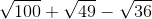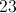Explanation:

Find the individual square roots and perform the operations on them.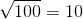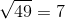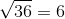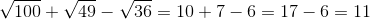### Example Question #1 : Squares / Square Roots

The square root of a number is 43. What is that number?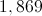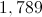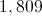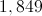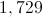Explanation:

By definition, the square root of a number multiplied by itself yields that number. Therefore, 43 is the square root of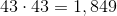.

### Example Question #2 : Squares / Square Roots

The square root of a number is 58. What is that number?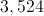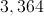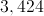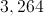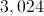Explanation:

By definition, the square root of a number multiplied by itself yields that number. Therefore,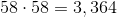.

### Example Question #3 : Squares / Square Roots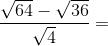Explanation:

First, find the square root of each number: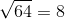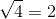Then, solve the equation accordingly.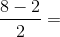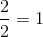The answer is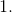### Example Question #2 : Squares / Square Roots

Evaluate: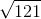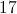Explanation:

The square root of a number is the number which, when multiplied by itself, yields that number. Since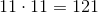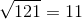.

### Example Question #3 : Squares / Square Roots

Which of the following statements is true about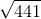?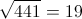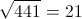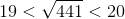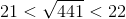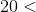Explanation:

The square root of a number is the number which, when multiplied by itself, yields that number. Since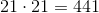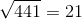.

### Example Question #7 : Squares / Square Roots

Which of the following statements is true about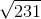?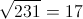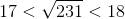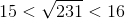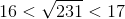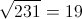Explanation:

The square root of a number is the number which, when multiplied by itself, yields that number.  Since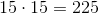,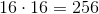, and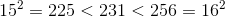then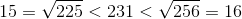### Example Question #1 : How To Find The Square Root

Evaluate: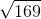Explanation:

The square root of a number is the number which, when multiplied by itself, yields that number. Since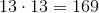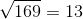.

### Example Question #4 : Squares / Square Roots

Which of the following statements is true about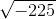?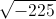is undefined in the set of real numbers.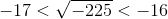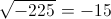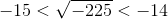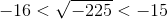is undefined in the set of real numbers.

Explanation:

A negative number does not have a real square root, so this is the correct choice.

### Example Question #2 : How To Find The Square Root

A square has an area of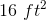.

How long is each of its sides?The two sides of the square must be the same length, and multiply to give 16.  So we are looking for the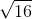which is.